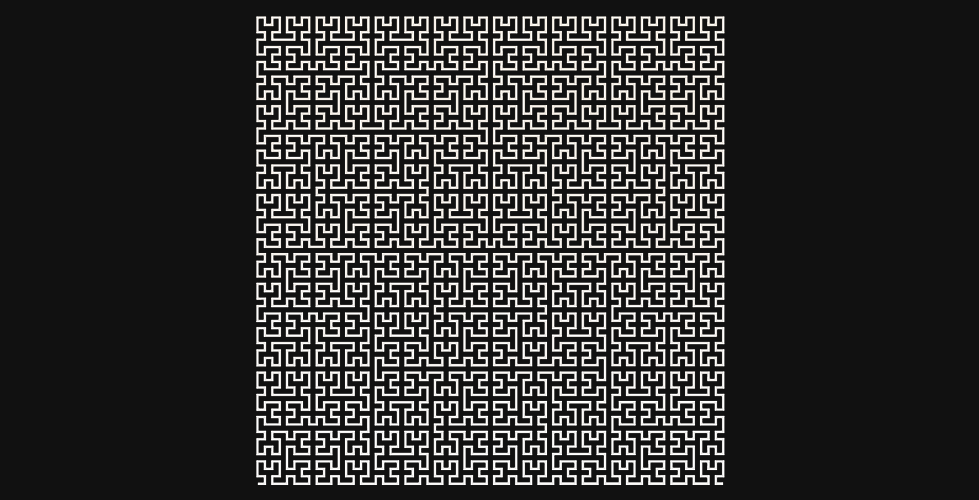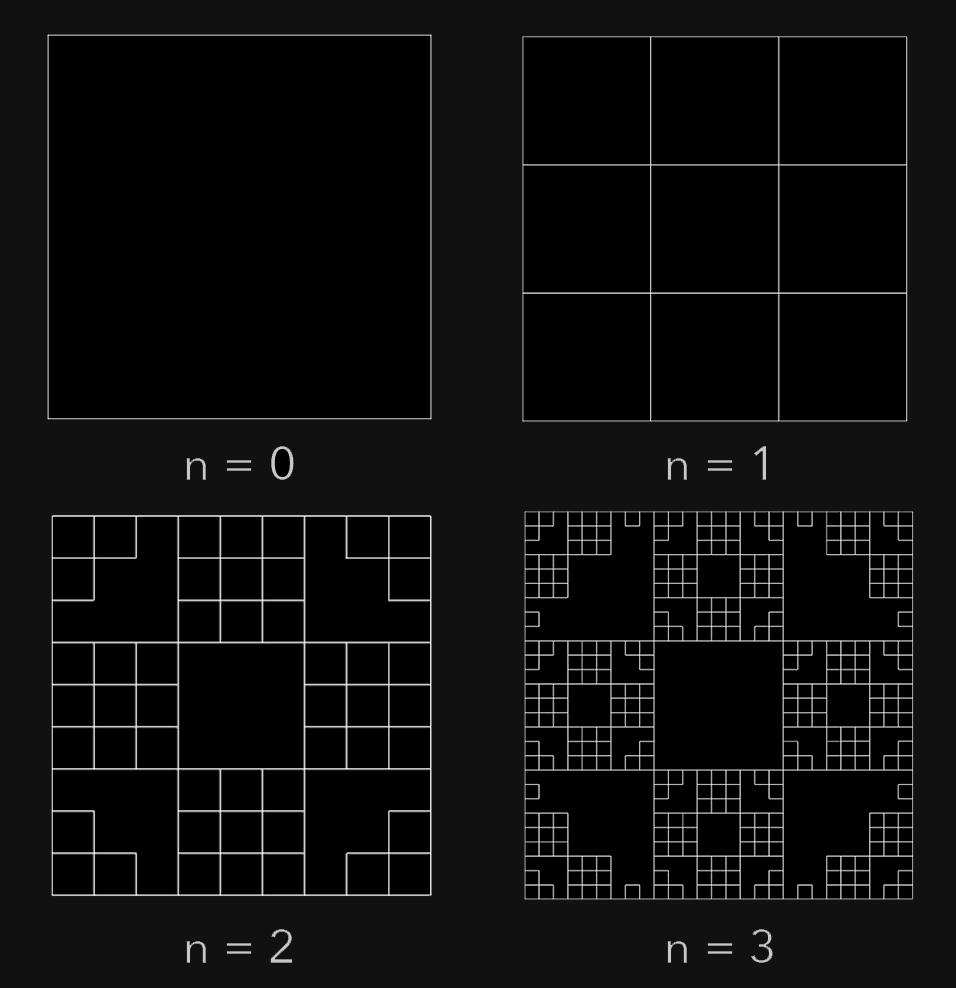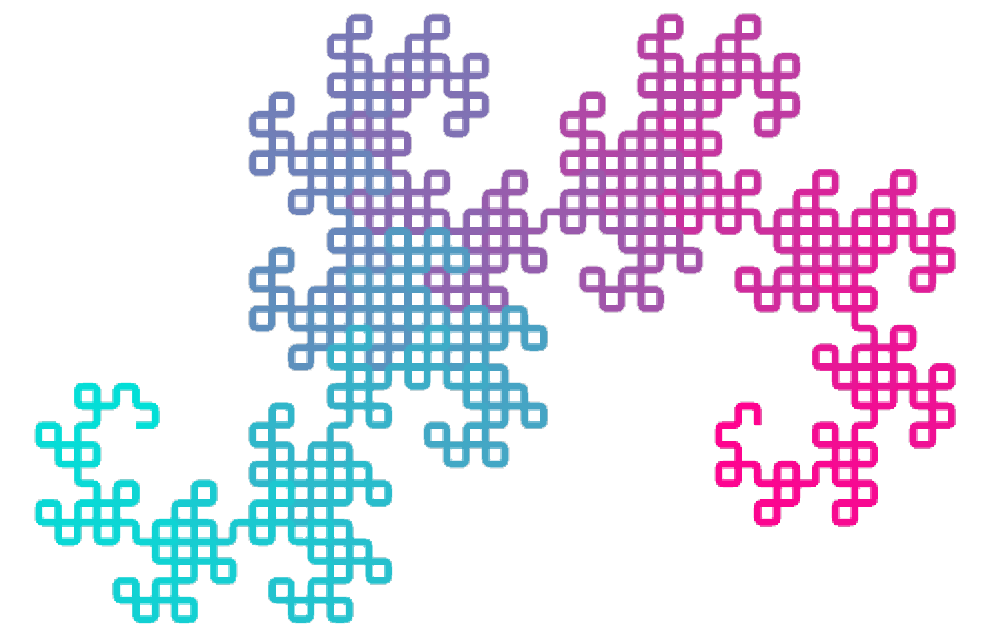## Overview

This post reviews the concept of non-branching L-systems and provides an approach for representing them in Unity, and drawing them in 2 different ways. Line Renderer and OpenGL Lines.

I’ve been reading The Algorithmic Beauty of Plants, which got me interested in the topic.## Drawing L-Systems

The concept of L-systems is: Begin with a start string (axiom). Apply a set of rewriting rules to each character (if applicable) to create a new string. Do this any number of iterations to create a final axiom. Then, interpret the characters in order as a series of drawing rules.

If we wanted to interpret an axiom, we’d have to prescribe meaning to each of the characters. Lets use

• F = “Draw forward”.
• - = “Rotate the forward vector by -90 degrees”;
• + = “Rotate the forward vector by 90 degrees”; (although unused in the first axiom) Given the axiom F-F-F-F, drawing based on the rules will generate a square.

Lets define the rewriting rule to generate a curve similar to a menger sponge.

``````F->FF-F-F-F-FF
``````

This means, replace every `F` in our axiom with the string `FF-F-F-F-FF`.

After 1 iteration, the string `F-F-F-F` will become:

``````FF-F-F-F-FF-FF-F-F-F-FF-FF-F-F-F-FF-FF-F-F-F-FF
``````By interpreting the string based on the draw rules above, here’s what the square deforms to after some iterations:

Drawing Different sets of drawing and replacement rules produce different shapes.

Implementation To represent a replacement rule, we will use a class to represent a character, and a corresponding replacement string.

Similar for a draw rule. A character with a corresponding angle. If there’s no angle, we assume it means to draw forward.

We will reference these from our LSystem class, which will have lists of each rule we can populate in the inspector.

The product of the LSystem class will be the positions array, so a line drawing class can handle those. To compute the positions, it first must generate the final axiom, and then iterate over the string, applying draw rules.

Two dictionaries are created on awake, replacementRuleDict and drawRuleDict to store character mappings for their rules. This makes it simple to lookup a rule for any given character.

I took a simple approach by just creating an empty string and iterating over the axiom, populating the string with rather the replacement rule for the current character, or the current character if no rule applies.

The code for calculating the positions isn’t that exciting.

It has a forward vector which is rotates when it encounters a draw rule in the axiom that has an angle. If the draw rule for the current character doesn’t have an angle, we assume that means to go forward, so we add forward to the current position, and add it to the list.

Line renderers are one approach to draw them. The LSystemsLineRenderer class just sets the positions and the code is trivial.

With many points, this can get pretty slow. A faster way to draw lines in Unity is to use OpenGL GL_Lines. These come with less features though, so it’s a trade off.Conclusion You can check out my LSystems implementation and sample project on Github

This approach is rather limiting, because it only handles continuous space filling curves. I’d like to experiment in the future with branching systems and axial trees.